# Triangle inequality - math problems

In any triangle, the sum of the lengths of any two sides is greater than the length of the remaining third one. The triangle inequality is three inequalities that are true simultaneously. The inequalities result directly from the triangle's construction. If one side were longer than two in total, the vertex against the longest side could not be constructed (or drawn), and the triangle as a shape in the plane would not exist.

#### Number of problems found: 11

• Perimeter of a triangle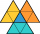If the perimeter of a triangle is 6 2/3 cm and the lengths of two of its sides are 2 1/2 cm and 3 1/3 cm, find the length if the third side
• Draw a triangle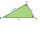We have line segments with lengths of 3cm, 5cm, 6cm, 7cm and 9cm. What is the probability in % that if I randomly select three of them I will be able to draw a triangle?
• Sin cos tan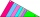In triangle ABC, right-angled at B. Sides/AB/=7cm, /BC/=5cm, /AC/=8.6cm. Find to two decimal places. A. Sine C B. Cosine C C. Tangent C.
• The perimeter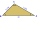The triangle has one side 5 cm long and the another 11 cm long. What can be the smallest and what is the largest perimeter?
• Possible lengths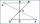Find the most possible lengths for the third side of a triangle with sides 20 and 18.
• Triangles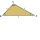Five sticks with a length of 2,3,4,5,6 cm. How many ways can you choose three sticks to form three sides of a triangle?
• Triangles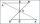Hanka cut the 20 cm long straws into three pieces each piece had a length in cm. Then, with these three pieces, she tried to make a triangle. a) What circuit has each of the triangles? b) How long can the longest side measure? c) How many different triang
• TrianglesIvo wants to draw all the triangles whose two sides of which have a length of 4 cm and 9 cm, and the length of the third side is expressed in whole centimeters. How many triangles does he have?
• Right trianglesHow many right triangles we can construct from line segments 3,4,5,6,8,10,12,13,15,17 cm long? (Do not forget to the triangle inequality).
• TriangleProve whether you can construct a triangle ABC, if a=9 cm, b=6 cm, c=10 cm.
• 3-bracket 2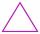May be the smallest angle in the triangle greater than 70°?

We apologize, but in this category are not a lot of examples.
Do you have an exciting math question or word problem that you can't solve? Ask a question or post a math problem, and we can try to solve it.

We will send a solution to your e-mail address. Solved examples are also published here. Please enter the e-mail correctly and check whether you don't have a full mailbox.